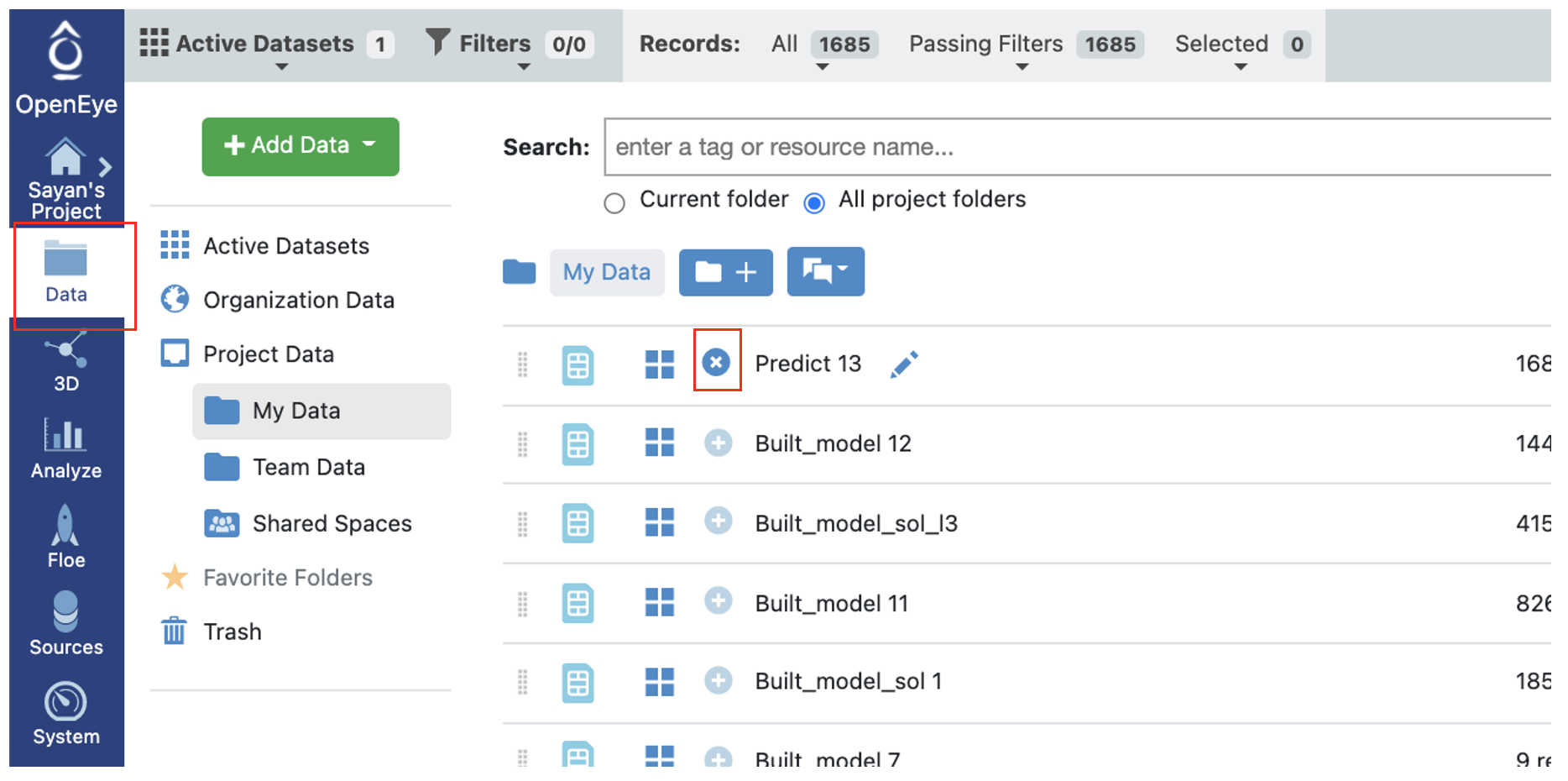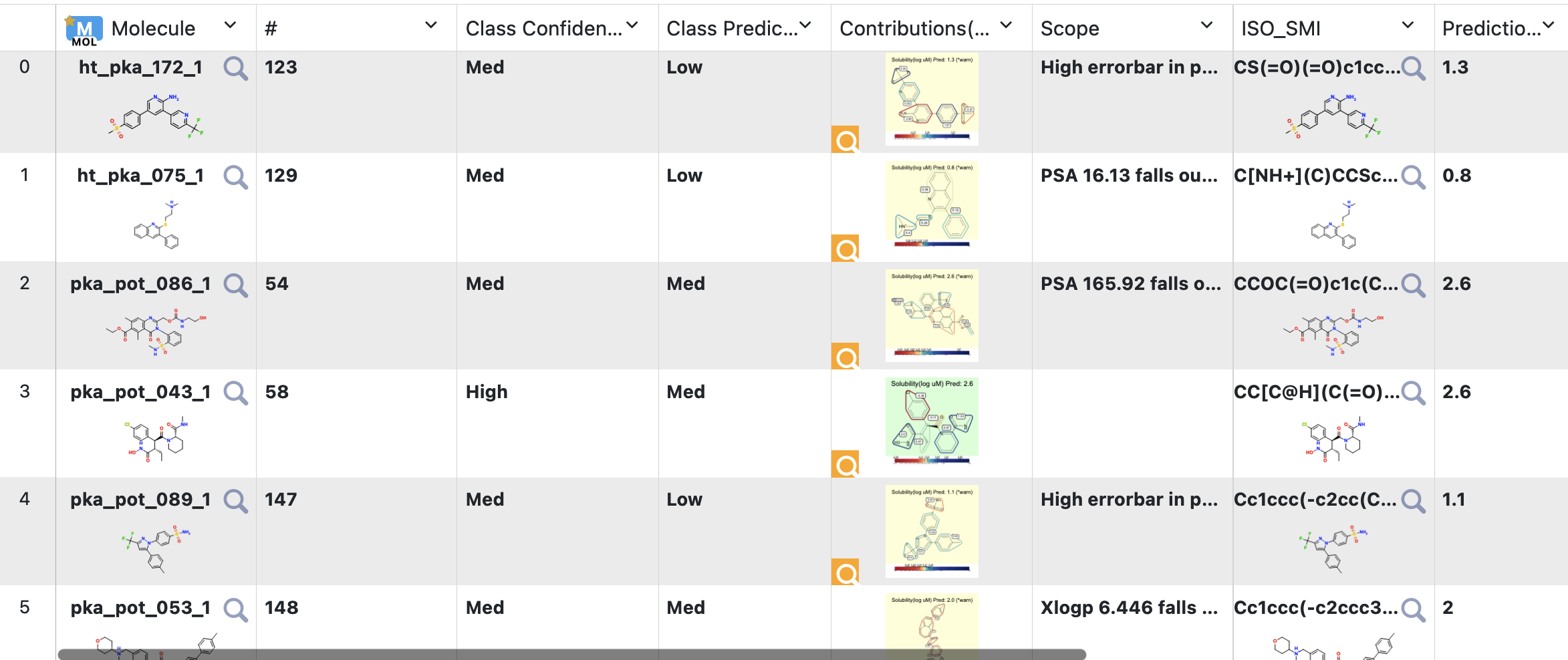# Tutorial: Use Pretrained Regression Fingerprint Model to Predict Property of Molecules¶

OpenEye Model Building is a tool to build machine learning models that predicts molecular properties.

In this tutorial, we will use a trained fully connected neural network model to predict physical property of molecules. The Floe predicts the property of each molecule and explans it. It also generate confidence intervals and other info. The Floe report provides a summary of the output.

This tutorial uses the following Floe:

• ML Regression using Fingerprints for Small Molecules

It is suggested to read the previous tutorial to learn how to build a machine learning model to use for prediction.

## Create a Tutorial Project¶

Note

If you have already created a Tutorial project you can re-use the existing one.

Log into Orion and click the home button at the top of the blue ribbon on the left of the Orion Interface. Then click on the ‘Create New Project’ button and in the pop up window enter Tutorial for the name of the project and click ‘Save’.

## Floe Input¶

The inputs required are:

• Molecule Dataset (P_1)

• Tensorflow Machine Learning Model Dataset (M_1)

The P_1 dataset contains several OERecord (s). The Floe expects an OEMol from each record. This is the molecules the model will predict physical property of. Let this dataset be P_1.

Here is a sample record from the dataset:

```OERecord (
Molecule(Chem.Mol) : c1ccc(c(c1)NC(=O)N)OC[C@H](CN2CCC3(CC2)Cc4cc(ccc4O3)Cl)O
)
```

There can be another float field containing the physical property values to validate against. The following dataset does not contain said field.

The M_1 dataset contains one or more machine learning models. To learn how to generate these models, read the previous tutorial on building models in Orion. We attach a sample trained Tensorflow Model to predict Solubility. In addition, you can have a Tensorflow probability model for domain of application(DOA) and error bar analysis. Here are the sample trained model.

## Run Generic Property Prediction Floe¶

• Click on the ‘Floe’ button in the left menu bar

• Click on the ‘Floes’ tab

• Under Categories select Packages

• Select OpenEye Model Building

• Click on the ML Regression using Fingerprints for Small Molecules and a Job Form will pop up. Specify the following parameter settings in the Job Form.

• For the Input Tensorflow Model, choose M_1 from above. Make sure you have the ID of “Model ID of which Tensorflow Model to Use to Predict” to 1.

• For the Input Tensorflow Probability Model, choose T_1 from above. Again, make sure you have the ID of “Model ID of which Tensorflow Probabability (TFP) Model to Use to Predict” to 1.

• For the Input Small Molecule Dataset, choose P_1 from above

• All the molecules predicted will be saved to name in the field Output Property. Change it to something recognizable.

• Additionally if your small molecule dataset has a field containing the predicted value (sourced from elsewhere), you can turn the validation option on to get a statistical comparison between this value and the prediction.

That’s it ! Things should run, generate an output and a Floe report

## Analyze OEModel Floe Report¶

Here is a sample image of how the Floe report should look (Assuming you ran a fully connected neural network model like M_1):

The top part contains the hyperparameters on which the model was trained. Then we have histogram of the output prediction, and the confidence with each prediction. We also have a plot for confidence of prediction versus the actual output. These overall statistics help analyze the input molecules predicted.

We also have outlier prediction using:

Lastly, there is a link to a page under Interesting Molecules and it shows the annotated images that explain the outlier and central molecules.

## Analyze Output¶

• Go to the data section of Orion and Activate the data the Floe produced. This should have the same name you chose for the Output Prediction field of your Floe. The data can be activated by clicking on the small plus sign in a circle right next to it.• Now going to the analyze page in Orion, you should be able to see the molecules, their predicted pyrrolamide values, and the explanation of the output.

• There are three output ports: Success, Failure, and No Confidence.

Outputs Meaning

• Failure Data: (a) Molecule is too large or too small. or, (b) Molecule has an unknown atom.

• No Confidence Data: Molecule’s property falls out of scope of training set. In this case, the model predicts with no guarantees. Explainer image has a red background.

• Success Data: (a) Falls within scope; explainer has green background. (b) Falls at the edge of scope; explainer has yellow background.

The output columns and their explanations are:

• Class Confidence(Property Unit): High, Med or Low confidence in prediction.

• Class Prediction(Property Unit): High, Med or Low prediction of property. Depends on if the value lies within <25% (Low), >75%(High), or in between.

• Contributions: Explanation of prediction based on a local model. If the image has a red background, it means no confidence. If yellow, its a warning. Green means it falls within scope of the predictor. Based on the choice of molecule explainer (Fragment by default), different parts will be color annotated with blue denoting more contribution towards the physical property while red denotes the opposite.

• Scope: If there is an error or warning, what caused the issue.

• Prediction(Property Unit): Physical property prediction of the molecule.Note

We assign IDs (#) on each record molecule. This follows a linear ordering over all molecules. So if you activate all the successful, no confidence, and failure predictions, and sort them based on #, the order should be same as input.